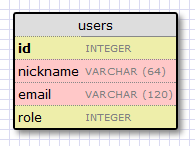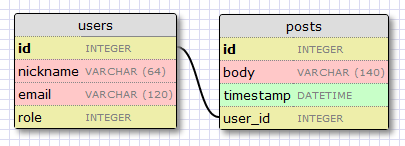# Flask 教程(4)：数据库

## 在Flask使用数据库

ORMs允许数据库程序用对象的方式替代表和SQL语句。面向对象的操作被ORM转化为数据库命令。这样就意味着，不用sql语句，让Flask-SQLAlchemy为我们执行sql语句。

## 迁移

pip install Flask-SQLALchemy

pip install sqlalchemy-migrate

## 配置

 1 2 3 4 5 `import` `os` `basedir ``=` `os.path.abspath(os.path.dirname(__file__))` `SQLALCHEMY_DATABASE_URI ``=` `'sqlite:///'` `+` `os.path.join(basedir, ``'app.db'``)` `SQLALCHEMY_MIGRATE_REPO ``=` `os.path.join(basedir, ``'db_repository'``)` SQLALCHEMY_TRACK_MODIFICATIONS = True  //这个在新版本要加上

SQLALCHEMY_DATABASE_URI是the Flask-SQLAlchemy必需的扩展。这是我们的数据库文件的路径。

SQLALCHEMY_MIGRATE_REPO 是用来存储SQLAlchemy-migrate数据库文件的文件夹。

 1 2 3 4 5 6 7 8 `from` `flask ``import` `Flask` `from` `flask.ext.sqlalchemy ``import` `SQLAlchemy` `app ``=` `Flask(__name__)` `app.config.from_object(``'config'``)` `db ``=` `SQLAlchemy(app)` `from` `app ``import` `views, models`

## 据库模型id字段通常作为主键的形式用在所有的models里面，每个在数据库中的user都有一个指定的唯一id值。幸运的是，这些都是自动的，我们只需要提供一个id字段。

nickname和email字段被定义为string类型，他们的长度也已经被指定，这样可以节省数据库存储空间。

role字段被定义为integer类型，我们用来标识users是admins还是其他类型。

 1 2 3 4 5 6 7 8 9 10 11 12 13 `from` `app ``import` `db` `ROLE_USER ``=` `0` `ROLE_ADMIN ``=` `1` `class` `User(db.Model):` `    ``id` `=` `db.Column(db.Integer, primary_key ``=` `True``)` `    ``nickname ``=` `db.Column(db.String(``64``), index ``=` `True``, unique ``=` `True``)` `    ``email ``=` `db.Column(db.String(``120``), index ``=` `True``, unique ``=` `True``)` `    ``role ``=` `db.Column(db.SmallInteger, default ``=` `ROLE_USER)` `    ``def` `__repr__(``self``):` `        ``return` `''` `%` `(``self``.nickname)`

User类把我们刚刚创建的几个字段定义为类变量。字段使用db.Column类创建实例，字段的类型作为参数，另外还提供一些其他可选参数。例如，标识字段唯一性和索引的参数.

__repr__方法告诉Python如何打印class对象，方便我们调试使用。

## 创建数据库

 1 2 3 4 5 6 7 8 9 10 11 12 `#!flask/bin/python` `from` `migrate.versioning ``import` `api` `from` `config ``import` `SQLALCHEMY_DATABASE_URI` `from` `config ``import` `SQLALCHEMY_MIGRATE_REPO` `from` `app ``import` `db` `import` `os.path` `db.create_all()` `if` `not` `os.path.exists(SQLALCHEMY_MIGRATE_REPO):` `    ``api.create(SQLALCHEMY_MIGRATE_REPO, ``'database repository'``)` `    ``api.version_control(SQLALCHEMY_DATABASE_URI, SQLALCHEMY_MIGRATE_REPO)` `else``:` `    ``api.version_control(SQLALCHEMY_DATABASE_URI, SQLALCHEMY_MIGRATE_REPO, api.version(SQLALCHEMY_MIGRATE_REPO))`

`./db_create.py`

## 第一次迁移

 1 2 3 4 5 6 7 8 9 10 11 12 13 14 15 `#!flask/bin/python` `import` `imp` `from` `migrate.versioning ``import` `api` `from` `app ``import` `db` `from` `config ``import` `SQLALCHEMY_DATABASE_URI` `from` `config ``import` `SQLALCHEMY_MIGRATE_REPO` `migration ``=` `SQLALCHEMY_MIGRATE_REPO ``+` `'/versions/%03d_migration.py'` `%` `(api.db_version(SQLALCHEMY_DATABASE_URI, SQLALCHEMY_MIGRATE_REPO) ``+` `1``)` `tmp_module ``=` `imp.new_module(``'old_model'``)` `old_model ``=` `api.create_model(SQLALCHEMY_DATABASE_URI, SQLALCHEMY_MIGRATE_REPO)` exec(old_model ,tmp_module.__dict__)  //新版本的写法 `script ``=` `api.make_update_script_for_model(SQLALCHEMY_DATABASE_URI, SQLALCHEMY_MIGRATE_REPO, tmp_module.meta, db.metadata)` `open``(migration, ``"wt"``).write(script)` `a ``=` `api.upgrade(SQLALCHEMY_DATABASE_URI, SQLALCHEMY_MIGRATE_REPO)` `print` `'New migration saved as '` `+` `migration` `print` `'Current database version: '` `+` `str``(api.db_version(SQLALCHEMY_DATABASE_URI, SQLALCHEMY_MIGRATE_REPO))`

`./db_migrate.py`

`New migration saved as db_repository/versions/001_migration.py  Current database version: 1`

## 数据库的升级和回滚 （这个我没试验）

 1 2 3 4 5 6 `#!flask/bin/python` `from` `migrate.versioning ``import` `api` `from` `config ``import` `SQLALCHEMY_DATABASE_URI` `from` `config ``import` `SQLALCHEMY_MIGRATE_REPO` `api.upgrade(SQLALCHEMY_DATABASE_URI, SQLALCHEMY_MIGRATE_REPO)` `print` `'Current database version: '` `+` `str``(api.db_version(SQLALCHEMY_DATABASE_URI, SQLALCHEMY_MIGRATE_REPO))`

 1 2 3 4 5 6 7 `#!flask/bin/python` `from` `migrate.versioning ``import` `api` `from` `config ``import` `SQLALCHEMY_DATABASE_URI` `from` `config ``import` `SQLALCHEMY_MIGRATE_REPO` `v ``=` `api.db_version(SQLALCHEMY_DATABASE_URI, SQLALCHEMY_MIGRATE_REPO)` `api.downgrade(SQLALCHEMY_DATABASE_URI, SQLALCHEMY_MIGRATE_REPO, v ``-` `1``)` `print` `'Current database version: '` `+` `str``(api.db_version(SQLALCHEMY_DATABASE_URI, SQLALCHEMY_MIGRATE_REPO))`

## 数据库关联posts表包含一个必须的id,微博的内容body，还有一个时间戳。没有什么新东西，但是user_id字段值得解释下。

 1 2 3 4 5 6 7 8 9 10 11 12 13 14 15 16 17 18 19 20 21 22 23 `from` `app ``import` `db` `ROLE_USER ``=` `0` `ROLE_ADMIN ``=` `1` `class` `User(db.Model):` `    ``id` `=` `db.Column(db.Integer, primary_key ``=` `True``)` `    ``nickname ``=` `db.Column(db.String(``64``), unique ``=` `True``)` `    ``email ``=` `db.Column(db.String(``120``), unique ``=` `True``)` `    ``role ``=` `db.Column(db.SmallInteger, default ``=` `ROLE_USER)` `    ``posts ``=` `db.relationship(``'Post'``, backref ``=` `'author'``, lazy ``=` `'dynamic'``)` `    ``def` `__repr__(``self``):` `        ``return` `''` `%` `(``self``.nickname)` `class` `Post(db.Model):` `    ``id` `=` `db.Column(db.Integer, primary_key ``=` `True``)` `    ``body ``=` `db.Column(db.String(``140``))` `    ``timestamp ``=` `db.Column(db.DateTime)` `    ``user_id ``=` `db.Column(db.Integer, db.ForeignKey(``'user.id'``))` `    ``def` `__repr__(``self``):` `        ``return` `''` `%` `(``self``.body)`

`./db_migrate.py`

`New migration saved as db_repository/versions/002_migration.py  Current database version: 2`

## 应用实践

`flask/bin/python`

Windows下在项目所在的根目录下，输入python，启动python

`flask/Scripts/python`

`>>> from app import db, models >>>`

 1 2 3 4 `>>> u ``=` `models.User(nickname``=``'john'``, email``=``'john@email.com'``, role``=``models.ROLE_USER)` `>>> db.session.add(u)` `>>> db.session.commit()` `>>>`

 1 2 3 4 `>>> u ``=` `models.User(nickname``=``'susan'``, email``=``'susan@email.com'``, role``=``models.ROLE_USER)` `>>> db.session.add(u)` `>>> db.session.commit()` `>>>`

 1 2 3 4 5 6 7 8 9 `>>> users ``=` `models.User.query.``all``()` `>>> ``print` `users` `[, ]` `>>> ``for` `u ``in` `users:` `...     ``print` `u.``id``,u.nickname` `...` `1` `john` `2` `susan` `>>>`

 1 2 3 4 `>>> u ``=` `models.User.query.get(``1``)` `>>> ``print` `u` `` `>>>`

 1 2 3 4 5 `>>> ``import` `datetime` `>>> u ``=` `models.User.query.get(``1``)` `>>> p ``=` `models.Post(body``=``'my first post!'``, timestamp``=``datetime.datetime.utcnow(), author``=``u)` `>>> db.session.add(p)` `>>> db.session.commit()`

 1 2 3 4 5 6 7 8 9 10 11 12 13 14 15 16 17 18 19 20 21 22 23 24 25 `# get all posts from a user` `>>> u ``=` `models.User.query.get(``1``)` `>>> ``print` `u` `` `>>> posts ``=` `u.posts.``all``()` `>>> ``print` `posts` `[]` `# obtain author of each post` `>>> ``for` `p ``in` `posts:` `...     ``print` `p.``id``,p.author.nickname,p.body` `...` `1` `john my first post!` `# a user that has no posts` `>>> u ``=` `models.User.query.get(``2``)` `>>> ``print` `u` `` `>>> ``print` `u.posts.``all``()` `[]` `# get all users in reverse alphabetical order` `>>> ``print` `models.User.query.order_by(``'nickname desc'``).``all``()` `[, ]` `>>>`

 1 2 3 4 5 6 7 8 9 10 `>>> users ``=` `models.User.query.``all``()` `>>> ``for` `u ``in` `users:` `...     db.session.delete(u)` `...` `>>> posts ``=` `models.Post.query.``all``()` `>>> ``for` `p ``in` `posts:` `...     db.session.delete(p)` `...` `>>> db.session.commit()` `>>>`